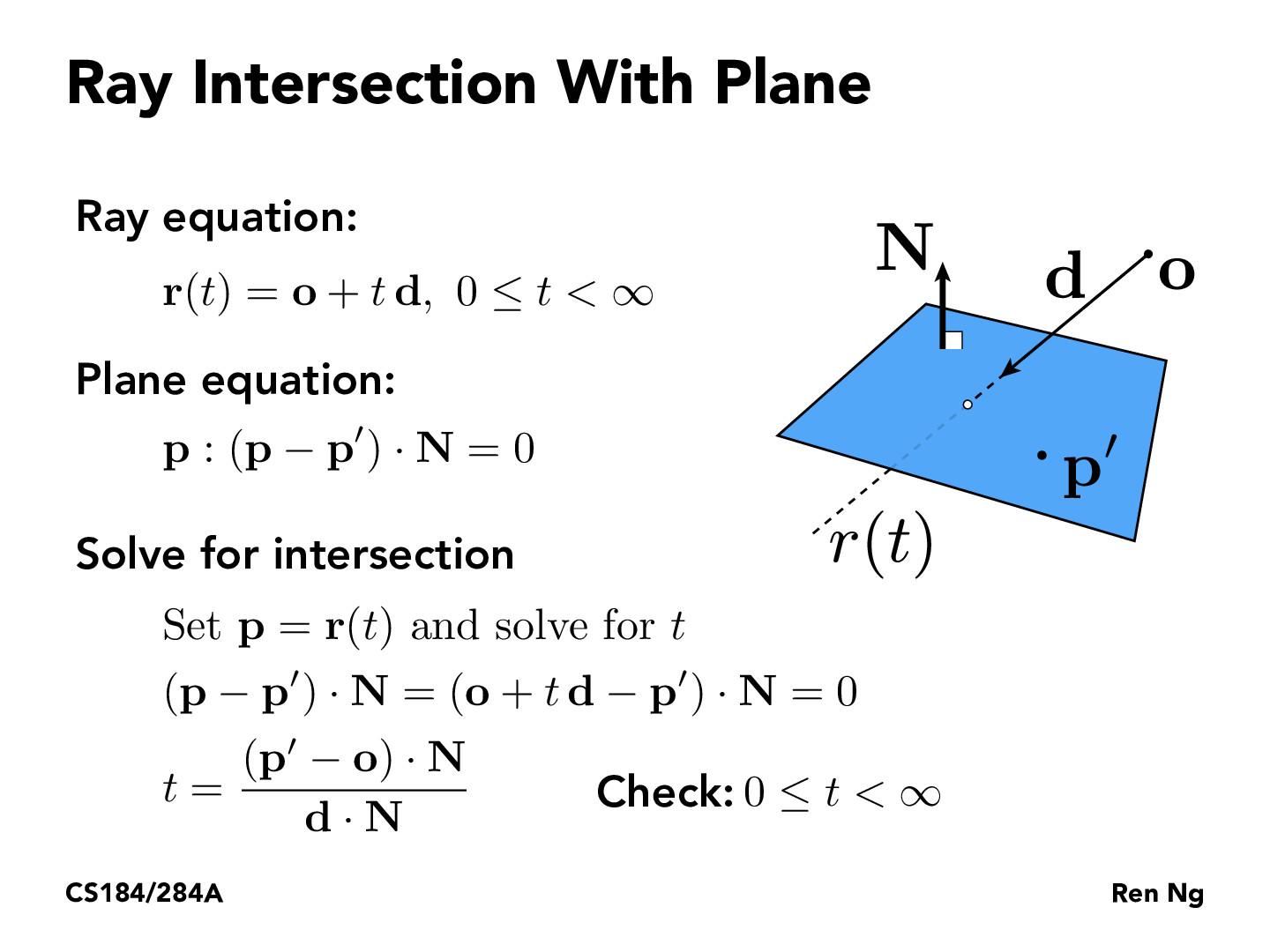You are viewing the course site for a past offering of this course. The current offering may be found here.Lecture 10: Ray Tracing (18)evan1997123

Can someone explain how they got the last step for solving t. I know Professor Ren said it's just moving parts, but I thought you couldn't do that if you had dot products.ellinzhao

$(\mathbf{o} - \mathbf{p}')\cdot \mathbf{N} = -t\mathbf{d}\cdot \mathbf{N}$

From here you can just divide out $\mathbf{d}\cdot \mathbf{N}$ because the result of the dot product is just a scalarziyaointl

Algebraically, $\mathbf{p}' \cdot \mathbf{N}$ should be cancelled on both sides, leaving the final equation as $t = \frac{(\mathbf{p}-\mathbf{o}) \cdot \mathbf{N}}{\mathbf{d} \cdot \mathbf{N}}$. However, since $\mathbf{p}$ and $\mathbf{p}'$ are just any two points on the plane, so I guess it doesn't matter.aninrusimha

A cool side note: If d is perpendicular with N, then the dot product of d and N is going to be 0. This means the result is undefined, which makes sense as it that case there are either 0 solutions (o is not on the plane) or infinite solutions

You must be enrolled in the course to comment